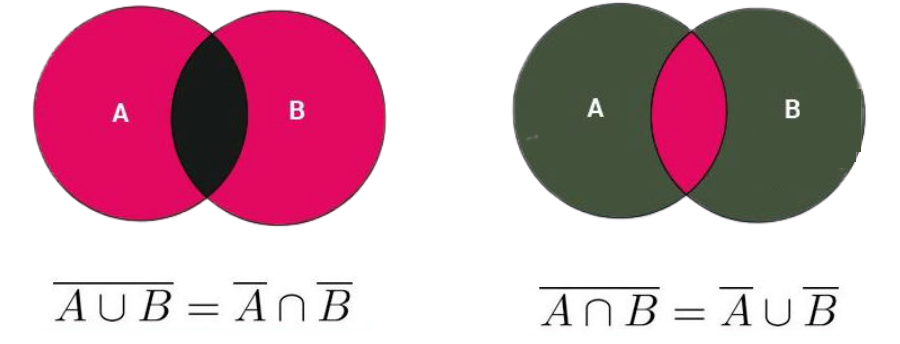# Properties of Complement Sets

De Morgan’s Law refers to the statement that the complement belonging to union of two Sets, Set A and Set B is equal to an intersection of two sets i.e. Set and Set B’s complement. As per De Morgan’s law, also, the intersection of two sets, Set A and Set B’s complement is equal to Union of complement of Set A and Set B, that is,

(A∪B)’ = A’ ∩ B’And (A ∩ B)’ = A’ ∪ B’

Set’s complement is described as following:

A’ = { x : x ∈ U & x ∉ A }

Where,

A’ = complement.## Properties of Complement of Sets:

i) Complement Laws: Set A’s Union and its complement, A’ delivers Universal Set U in which, A and A’ are the subset.

A ∪ A’ = U

Also, intersection in Set A in addition to its complement A’ provides the empty set “∅”

A ∩ A’ = ∅

Let’s Say: If U = {1 , 2 , 3 , 4 , 5 } then A = {4 , 5} then, A’ = {1 , 2 , 3 }. From this, you can observe that

A ∪ A’ = U = { 1 , 2 , 3 , 4 , 5}

Also,

A ∩ A’ = ∅

ii) Law of Double Complementation: As per this law, if you take complement of the complemented set A’ then, you’ll have set A itself, as a result.

(A’ )’ = A

In earlier example, you can observe that, if U = {1 , 2 , 3 , 4 , 5} and A = {4 , 5} then A’ = {1 , 2 , 3 }. Now, if you take complement of set A’, you get,

(A’ )’ = {4 , 5} = A

This gives you set A itself.

iii) Law of empty set and universal set:

As per this law, complement of Universal Set contributes in giving an empty set & vice-versa, that is,

∅’ = U And U = ∅’

This law is a self-explanatory one.## Some of the properties of complement sets

(i) A ∪ A’ = A’ ∪ A = ∪ – (Complement law)

(ii) (A ∩ B’) = ϕ – (Complement law)

(iii) (A ∪ B) = A’ ∩ B’ – (De Morgan’s first law)

(iv) (A ∩ B)’ = A’ ∪ B’ – (De Morgan’s second law)

(v) (A’)’ = A (Law of complementation)

(vi) ϕ’ = ∪ (Law of empty set

(vii) ∪’ = ϕ and universal set)

Now,

For any of the two finite sets; Set A and Set B;

(i) (A U B)’ = A’ ∩ B’ (which is De Morgan’s First Law or De Morgan’s Law of Union).

(ii) (A ∩ B)’ = A’ U B’ (which is De Morgan’s Second or De Morgan’s Law of Intersection).

## Proof of De Morgan’s law: (A U B)’ = A’ ∩ B’

Assuming:

P = (A U B)’,

Q = A’ ∩ B’,

Let us say that “x” be the arbitrary element of P, subsequently x ∈ P ⇒ x ∈ (A U B)’

– As x ∉ (A U B)

-therefore, x ∉ A and x ∉ B

– And with that, x ∈ A’ and x ∈ B’

– x ∈ A’ ∩ B’ (intersection of sets)

– x ∈ Q

Thus, P ⊂ Q …………….. Equation 1

Furthermore, let y be the arbitrary element of Q, resulting, y ∈ Q ⇒ y ∈ A’ ∩ B’

– y ∉ A and y ∉ B

– y ∉ (A U B)

– y ∈ A’ and y ∈ B’

– y ∈ (A U B)’

– y ∈ P

Accordingly, Q ⊂ P …………….. Equation 2

Now on combining equation 1 and equation 2, what we get is – P = Q, that is, (A U B)’ = A’ ∩ B’

Hence, De Morgan’s First Law gets verified!

## Proof of De Morgan’s law: (A ∩ B)’ = A’ U B’

Assuming,

M = (A ∩ B)’,

N = A’ U B’

Taking x as the arbitrary element of M, Next, x ∈ M ⇒ x ∈ (A ∩ B)’

With that:

As, x ∉ (A ∩ B)

So, either x ∉ A or x ∉ B

– When x ∈ A’ or x ∈ B’

– Then, x ∈ A’ U B’

⇒ x ∈ N

Thus, M ⊂ N …………….. Equation 1

In Addition, let y be the arbitrary element of N, then y ∈ N ⇒ y ∈ A’ U B’

y ∈ A’ or you can say y ∈ B’

– When, y ∉ A or y ∉ B

– Then, y ∉ (A ∩ B) (intersection)

⇒ y ∈ (A ∩ B)’ (complement of intersection)

⇒ y ∈ M

Thus, N ⊂ M …………….. (ii)

Now on combining equation 1 and equation 2, we get – M = , that is, (A ∩ B)’ = A’ U B’

Hence, De Morgan’s Second Law is verified!